• Unity3D模拟太阳系仿真 模拟要求 写一个程序，实现一个完整的太阳系， 其他星球围绕太阳的转速必须不一样，且不在一个法平面上。 操作步骤 1.创建如下结构 sun 里包括8大行星， 并且设置...
用Unity3D模拟太阳系仿真
模拟要求
写一个程序，实现一个完整的太阳系， 其他星球围绕太阳的转速必须不一样，且不在一个法平面上。
操作步骤
1.创建如下结构 sun 里包括8大行星， 并且设置好距离和大小
建立结构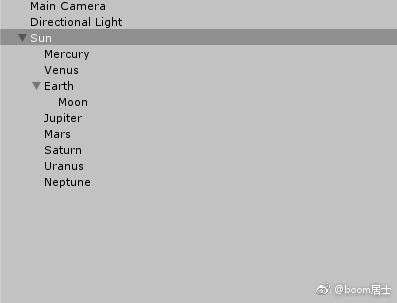建议用2D显示来直观设置距离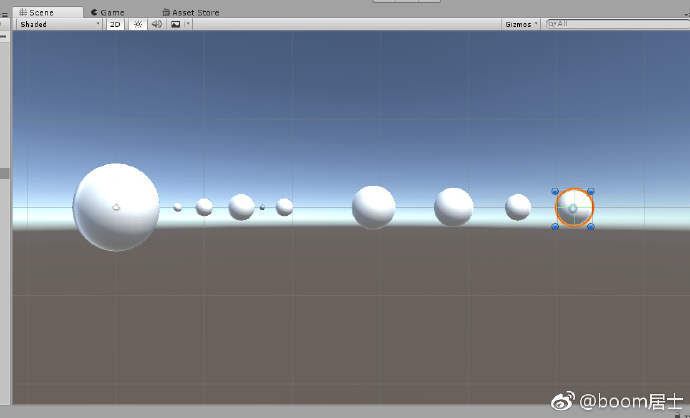2.在网上找到相应贴图 添加到assets
贴图网址
网址失效 素材更新链接如下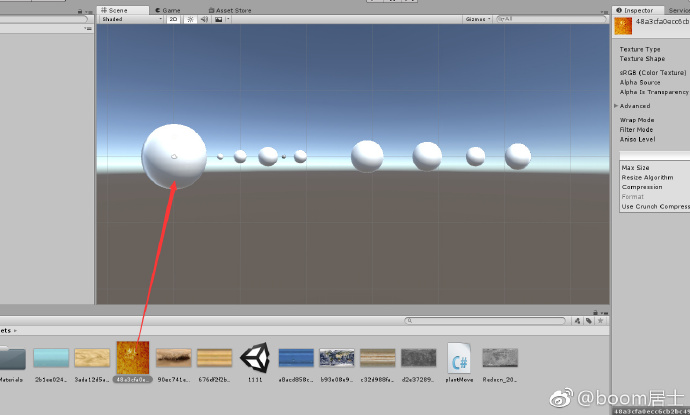而且把对应行星的贴图图片拖到对应的球体上（给白色小球上色）得到下面结果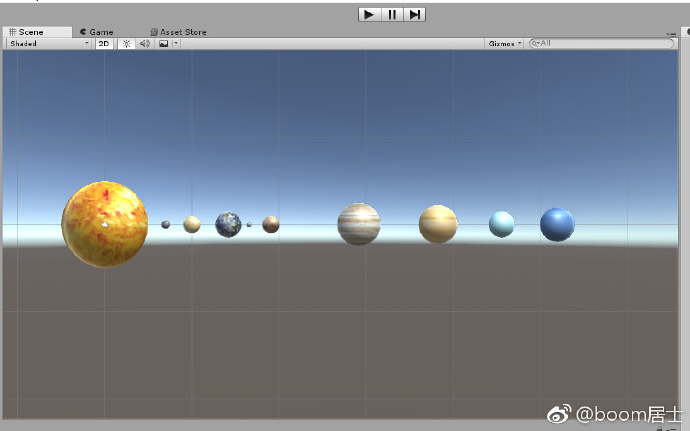3.创建c#脚本 使每个行星绕太阳转
创建plantMove.cs文件 代码如下
把此脚本拖到sun 物体即可，代码就是在sun 里面找到各个行星，并且设置运动
using System.Collections;
using System.Collections.Generic;
using UnityEngine;

public class plantMove : MonoBehaviour
{

// Use this for initialization
void Start()
{

}

// Update is called once per frame
void Update()
{

GameObject.Find("Sun").transform.Rotate(Vector3.up * Time.deltaTime * 5 );

GameObject.Find("Mercury").transform.RotateAround(Vector3.zero, new Vector3(0.1f, 1, 0), 60 * Time.deltaTime);
//设置公转的方向和速度  方向轴为（0， 1， 0） 速度为 60
GameObject.Find("Mercury").transform.Rotate(Vector3.up * Time.deltaTime * 10000 / 58);
//设置自转 自转速度为10000/58   58是水星的自传周期  倒数就是时间  下同

GameObject.Find("Venus").transform.RotateAround(Vector3.zero, new Vector3(0, 1, -0.1f), 55 * Time.deltaTime);
GameObject.Find("Venus").transform.Rotate(Vector3.up * Time.deltaTime * 10000 / 243);

GameObject.Find("Earth").transform.RotateAround(Vector3.zero, new Vector3(0, 1, 0), 50 * Time.deltaTime);
GameObject.Find("Earth").transform.Rotate(Vector3.up * Time.deltaTime * 10000);

GameObject.Find("Moon").transform.RotateAround(Vector3.zero, new Vector3(0, 1, 0), 5 * Time.deltaTime);
GameObject.Find("Moon").transform.Rotate(Vector3.up * Time.deltaTime * 10000/27);

GameObject.Find("Mars").transform.RotateAround(Vector3.zero, new Vector3(0.2f, 1, 0), 45 * Time.deltaTime);
GameObject.Find("Mars").transform.Rotate(Vector3.up * Time.deltaTime * 10000);

GameObject.Find("Jupiter").transform.RotateAround(Vector3.zero, new Vector3(-0.1f, 2, 0), 35 * Time.deltaTime);
GameObject.Find("Jupiter").transform.Rotate(Vector3.up * Time.deltaTime * 10000 / 0.3f);

GameObject.Find("Saturn").transform.RotateAround(Vector3.zero, new Vector3(0, 1, 0.2f), 20 * Time.deltaTime);
GameObject.Find("Saturn").transform.Rotate(Vector3.up * Time.deltaTime * 10000 / 0.4f);

GameObject.Find("Uranus").transform.RotateAround(Vector3.zero, new Vector3(0, 2, 0.1f), 15 * Time.deltaTime);
GameObject.Find("Uranus").transform.Rotate(Vector3.up * Time.deltaTime * 10000 / 0.6f);

GameObject.Find("Neptune").transform.RotateAround(Vector3.zero, new Vector3(-0.1f, 1, -0.1f), 10 * Time.deltaTime);
GameObject.Find("Neptune").transform.Rotate(Vector3.up * Time.deltaTime * 10000 / 0.7f);

}
}

代码解释：
我们通过GameObject.Find(“value”)来找到各个球体 然后通过调用RotateAround（）设置公转，通过调用Rotate（）方法设置自转
4. 进一步思考
运行下发现月球绕地球旋转不对劲， 其原因是地球的自转影响了月球的公转， 月球是相对一个自转的地球来的 月球公转叠加了地球自转。
所以怎么去处理这个问题呢？ 怎么让月球公转和地球自转不相关呢？
这里有一个解决方案
在原来的Sun里再加入一个对象名为EarthClone，而且大小和位置都和地球相同， 然后我对EarthClone设置和Earth一样的公转 但是不设置自转  把Moon放到EarthClone里让Moon相对与EarthClone公转 这样就能解决问题了
文件结构：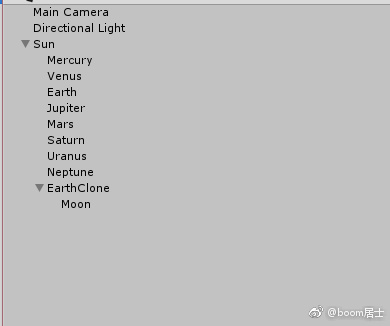加入和更改下列代码
 void Update()
{

GameObject.Find("Sun").transform.Rotate(Vector3.up * Time.deltaTime * 5 );

GameObject.Find("Mercury").transform.RotateAround(Vector3.zero, new Vector3(0.1f, 1, 0), 60 * Time.deltaTime);
GameObject.Find("Mercury").transform.Rotate(Vector3.up * Time.deltaTime * 1 / 58);

GameObject.Find("Venus").transform.RotateAround(Vector3.zero, new Vector3(0, 1, -0.1f), 55 * Time.deltaTime);
GameObject.Find("Venus").transform.Rotate(Vector3.up * Time.deltaTime * 10 / 243);

GameObject.Find("Earth").transform.RotateAround(Vector3.zero, new Vector3(0, 1, 0), 50 * Time.deltaTime);
GameObject.Find("Earth").transform.Rotate(Vector3.up * Time.deltaTime * 10);
//只设置公转 不设置自传
GameObject.Find("EarthClone").transform.RotateAround(Vector3.zero, new Vector3(0, 1, 0), 50 * Time.deltaTime);
//令Moon相对与不自转的EarthClone公转
GameObject.Find("Moon").transform.RotateAround(GameObject.Find("EarthClone").transform.position, new Vector3(0, 1, 0), 250 * Time.deltaTime);
GameObject.Find("Moon").transform.Rotate(Vector3.up * Time.deltaTime * 10/27);

GameObject.Find("Mars").transform.RotateAround(Vector3.zero, new Vector3(0.2f, 1, 0), 45 * Time.deltaTime);
GameObject.Find("Mars").transform.Rotate(Vector3.up * Time.deltaTime * 10);

GameObject.Find("Jupiter").transform.RotateAround(Vector3.zero, new Vector3(-0.1f, 2, 0), 35 * Time.deltaTime);
GameObject.Find("Jupiter").transform.Rotate(Vector3.up * Time.deltaTime * 10 / 0.3f);

GameObject.Find("Saturn").transform.RotateAround(Vector3.zero, new Vector3(0, 1, 0.2f), 20 * Time.deltaTime);
GameObject.Find("Saturn").transform.Rotate(Vector3.up * Time.deltaTime * 10 / 0.4f);

GameObject.Find("Uranus").transform.RotateAround(Vector3.zero, new Vector3(0, 2, 0.1f), 15 * Time.deltaTime);
GameObject.Find("Uranus").transform.Rotate(Vector3.up * Time.deltaTime * 10 / 0.6f);

GameObject.Find("Neptune").transform.RotateAround(Vector3.zero, new Vector3(-0.1f, 1, -0.1f), 10 * Time.deltaTime);
GameObject.Find("Neptune").transform.Rotate(Vector3.up * Time.deltaTime * 10 / 0.7f);

}


5.结果显示
把cs脚本拖到Sun对象里  运行查看结果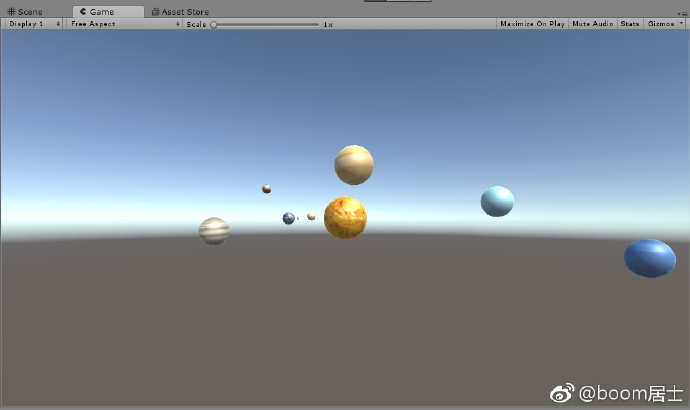展开全文Unity3D C#
• 简介这篇博客主要介绍了怎么简单的使用Unity3d来搭建一个简单的太阳系，以及做一个学习记录，分享一下学习Unity3d的心得。 使用工具：Unity3d 5.5实现1.材料的管理先是界面的选择吧，这里我选的是“2 by 3”的界面...
简介

这篇博客主要介绍了怎么简单的使用Unity3d来搭建一个简单的太阳系，以及做一个学习记录，分享一下学习Unity3d的心得。
使用工具：Unity3d 5.5

实现

1.材料的管理

先是界面的选择吧，这里我选的是“2 by 3”的界面，我觉得这个界面的好处就是，能尽可能的把所有的工作窗口显示出来吧。（其实是被同学安利的= =），至于怎么调，下图已说明：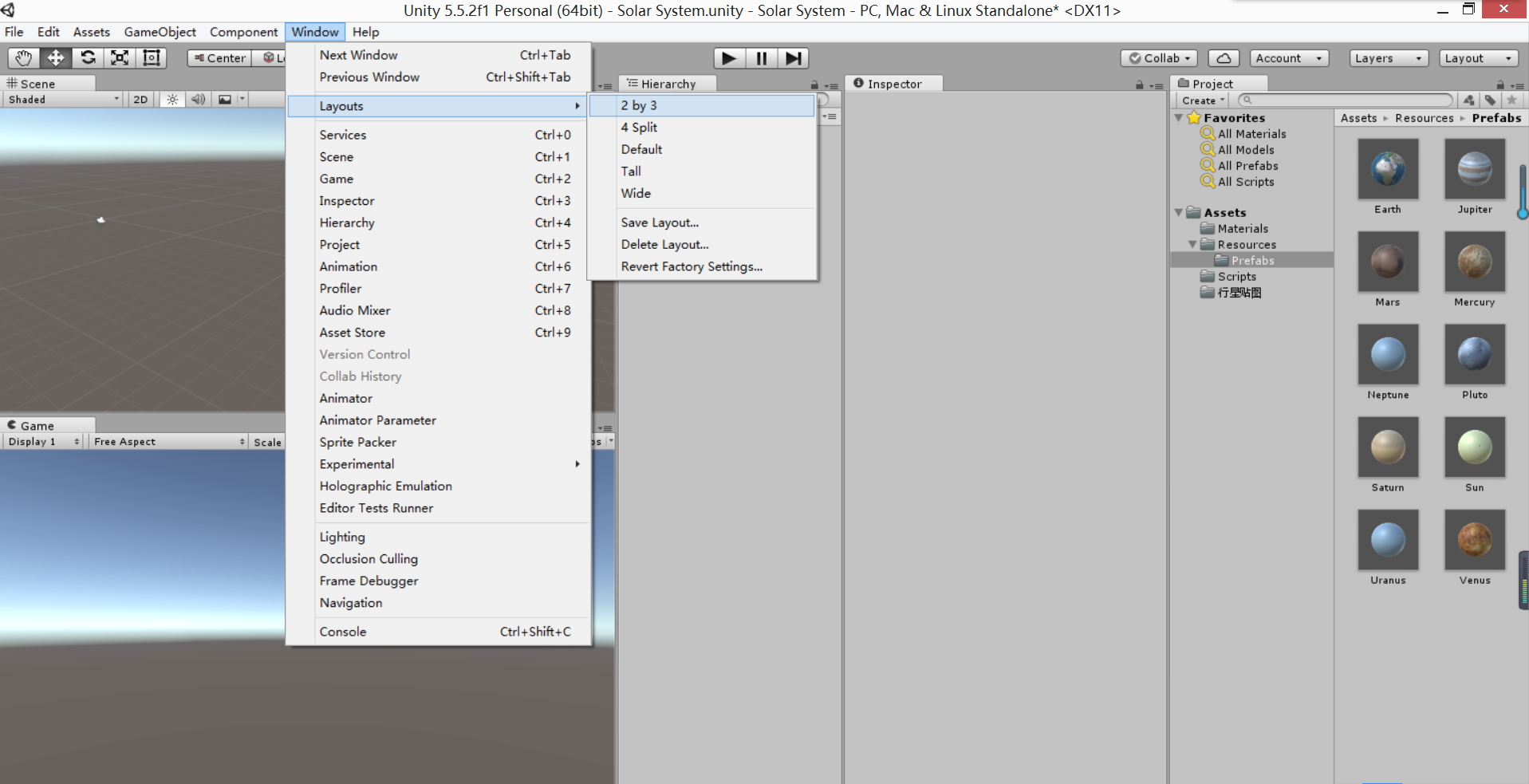由于行星大多数都是属于球体，所以我们要新建一个球，新建的方法如下：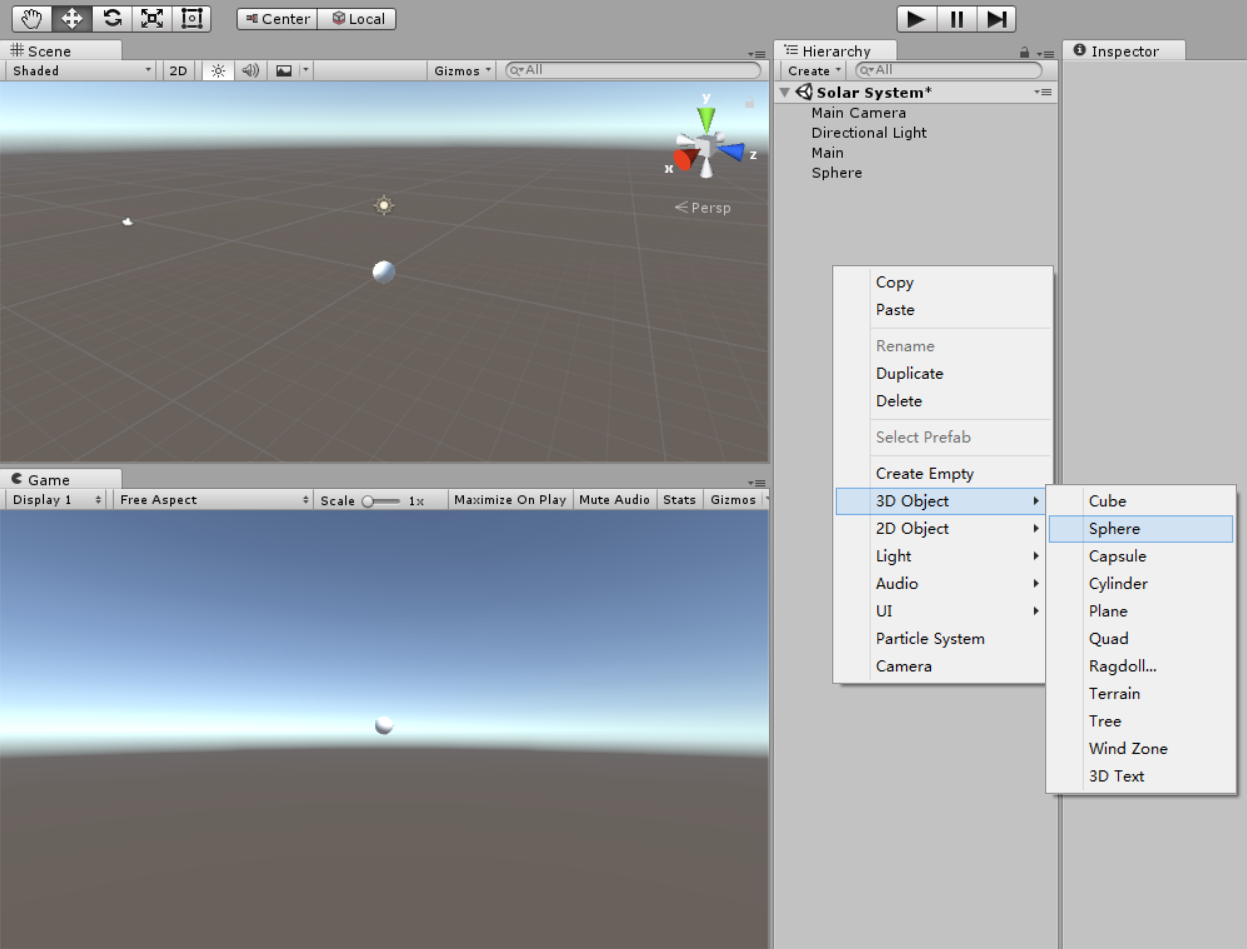新建完成之后，就可以在Scene界面跟Game界面见到这个球了。改名什么的也比较容易，在这就不说了。
然后就是将贴图贴在球上，让它看起来更加的真实。这里我选了地球来做例子（谁叫我是地球人呢（笑））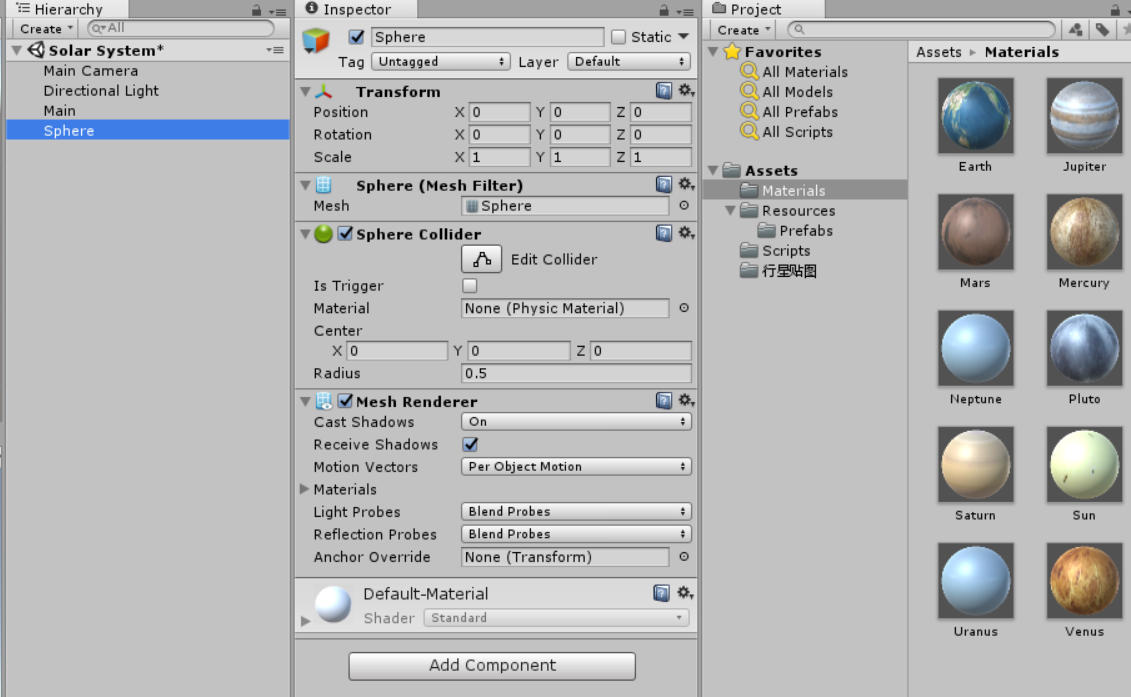在这个图片里，有几个属性是需要我们去了解的，先是Inspector，只要你点击了球的对象，那么Inspector就会有它的属性显示出来，这里就能改变它的属性，比如说Transform属性下的Position（位置），Rotation（转向），Scale（放大倍数）。至于其它属性，在此篇博客上没有用到，至于以后用到的时候，会说的。然后就是最右边用来表示该工程的文件的Assets文件夹，上课听了老师说，文件的存放最好是按照类别来存放，这样才不会使得文件类型变得混乱。一般就是Materials，Resources，Scripts三个文件夹，然后Materials是用来存放对象的材料的，而Resources就是用来存放资源，例如：Prefabs（预设）之类的东西，然后剩下的Scripts就是用来放置游戏脚本的。至于太阳系的Materials，在博客的最后面我会给出。
然后，你只需要把Materials里面的地球贴图拖到你要的球体上，你就得到一个地球（模型）啦。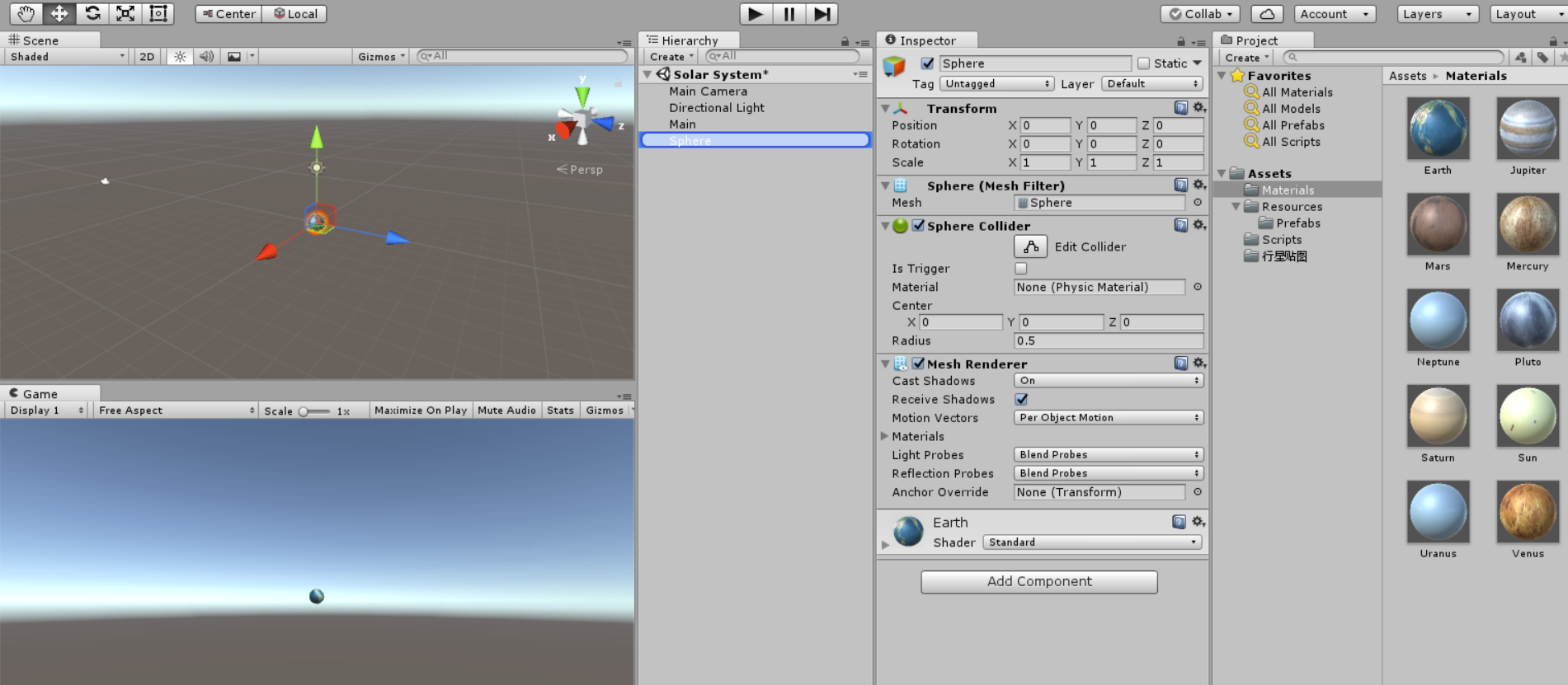然后，在这里要做一件重要的事情就是，你需要把你的地球模型，拖到Prefabs上，这是因为，当你的游戏做大之后，你会有许多的对象，假如你的对象都在Hierarchy上的话，这会使得对象十分的混乱，为了整洁，将其拖到Prefabs上是个很好的解决方法，Hierarchy窗口只留下一些比较重要的对象就行了。至于剩下的星球，重复前面的工作，就可以制作出所有的球体啦。
然后我们再创建一个空的对象，并把他命名为Main，用来搭载我们的游戏模型，以及脚本，而游戏对象的预制则由脚本生成，这样的管理方便我们的对象。然后把对象清除，保留预制就行了。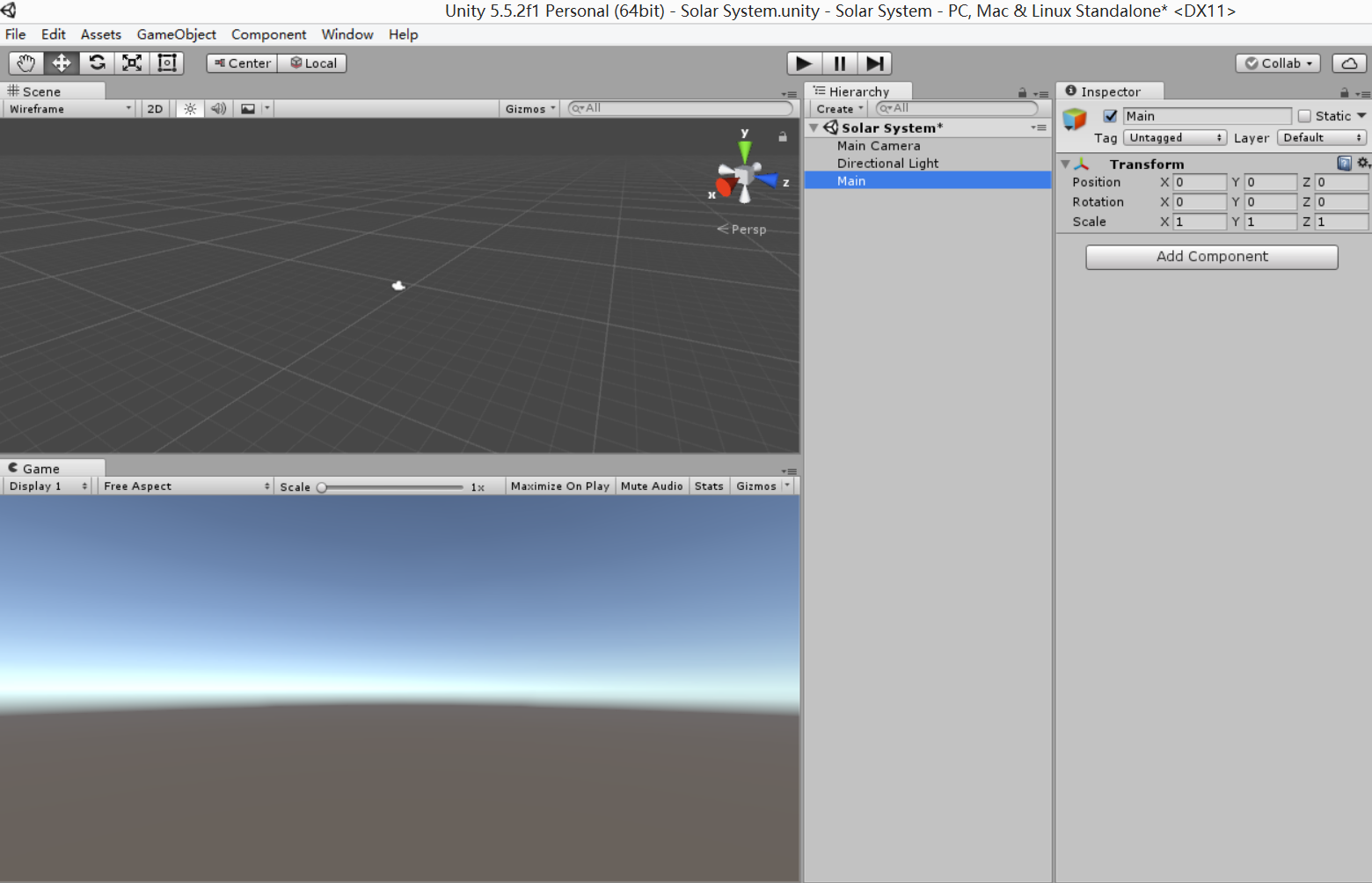界面是十分的简洁的，只有摄像机，灯光和控制游戏的脚本。
然后，我们就要编辑脚本了。

2.脚本的编辑

先在Scripts文件夹创建两个脚本文件，Controller跟Director，其实Unity的脚本可以用两种语言来写，分别是JavaScript跟C#，因为上课用的是C#，这里也就用C#来写了。

Director.cs

using System.Collections;
using System.Collections.Generic;
using UnityEngine;

public class Director : System.Object {

//singlton instance
private static Director _instance;

public ISceneController currentSceneController { get; set; }

// get instance anytime anywhere!
public static Director getInstance() {
if (_instance == null) {
_instance = new Director ();
}
return _instance;
}

public int getFPS() {
return Application.targetFrameRate;
}

public void setFPS(int fps) {
Application.targetFrameRate = fps;
}

public interface ISceneController {
void Pause();
void Resume();
}
}


Controller.cs

using System.Collections;
using System.Collections.Generic;
using UnityEngine;

public class Controller : MonoBehaviour, Director.ISceneController {

// the first scripts
void Awake() {
Director director = Director.getInstance ();
director.setFPS (60);
director.currentSceneController = this;
}
Transform Sun;
Transform Mercury;
Transform Venus;
Transform Earth;
Transform Mars;
Transform Jupiter;
Transform Saturn;
Transform Uranus;
Transform Neptune;
Sun = Instantiate<Transform> (
Vector3.zero, Quaternion.identity);
Mercury = Instantiate<Transform> (
new Vector3 (2, 0, 0), Quaternion.identity);
Venus = Instantiate<Transform> (
new Vector3 (4, 0, 0), Quaternion.identity);
Earth = Instantiate<Transform> (
new Vector3 (6, 0, 0), Quaternion.identity);
Mars = Instantiate<Transform> (
new Vector3 (8, 0, 0), Quaternion.identity);
Jupiter = Instantiate<Transform> (
new Vector3 (10, 0, 0), Quaternion.identity);
Saturn = Instantiate<Transform> (
new Vector3 (12, 0, 0), Quaternion.identity);
Uranus = Instantiate<Transform> (
new Vector3 (14, 0, 0), Quaternion.identity);
Neptune = Instantiate<Transform> (
new Vector3 (16, 0, 0), Quaternion.identity);

//named the sphere
Sun.name = "Sun";
Mercury.name = "Mercury";
Mercury.transform.parent = Sun.transform;
Venus.name = "Venus";
Venus.transform.parent = Sun.transform;
Earth.name = "Earth";
Earth.transform.parent = Sun.transform;
Mars.name = "Mars";
Mars.transform.parent = Sun.transform;
Jupiter.name = "Jupiter";
Jupiter.transform.parent = Sun.transform;
Saturn.name = "Saturn";
Saturn.transform.parent = Sun.transform;
Uranus.name = "Uranus";
Uranus.transform.parent = Sun.transform;
Neptune.name = "Neptune";
Neptune.transform.parent = Sun.transform;
}

// Use this for initialization
void Start() {
}

void Update() {
Mercury.RotateAround (Sun.position, new Vector3 (0, 1, 1), 1 * Time.deltaTime);
Mercury.Rotate (Vector3.up * 30 * Time.deltaTime);
Venus.RotateAround (Sun.position, new Vector3 (0, 2, 1), 2 * Time.deltaTime);
Venus.Rotate (Vector3.up * 30 * Time.deltaTime);
Earth.RotateAround (Sun.position, new Vector3 (0, 1, 2), 10 * Time.deltaTime);
Earth.Rotate (Vector3.up * 30 * Time.deltaTime);
Mars.RotateAround (Sun.position, new Vector3 (0, 1, 3), 4 * Time.deltaTime);
Mars.Rotate (Vector3.up * 30 * Time.deltaTime);
Jupiter.RotateAround (Sun.position, new Vector3 (0, 3, 1), 5 * Time.deltaTime);
Jupiter.Rotate (Vector3.up * 30 * Time.deltaTime);
Saturn.RotateAround (Sun.position, new Vector3 (0, 2, 3), 6 * Time.deltaTime);
Saturn.Rotate (Vector3.up * 30 * Time.deltaTime);
Uranus.RotateAround (Sun.position, new Vector3 (0, 3, 2), 7 * Time.deltaTime);
Uranus.Rotate (Vector3.up * 30 * Time.deltaTime);
Neptune.RotateAround (Sun.position, new Vector3 (0, 1, 5), 8 * Time.deltaTime);
Neptune.Rotate (Vector3.up * 30 * Time.deltaTime);
}

}



（这里我用的是Unity自带的MonoDevelop代码编辑器，比VS轻量，挺好用的，推荐编辑脚本的时候可以使用这个。）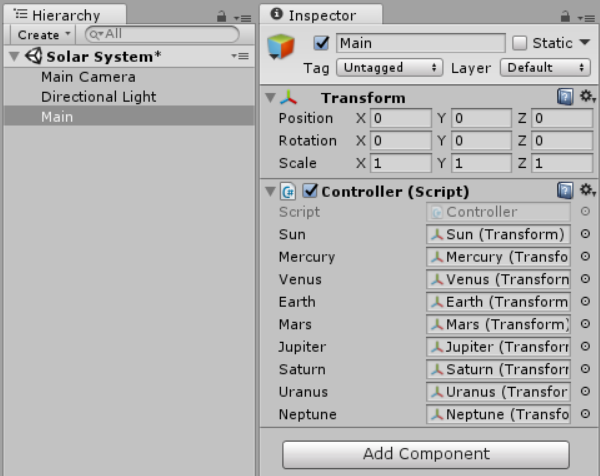调整调整灯光位置和照相机的位置，然后点运行，我们的太阳系就跑起来啦。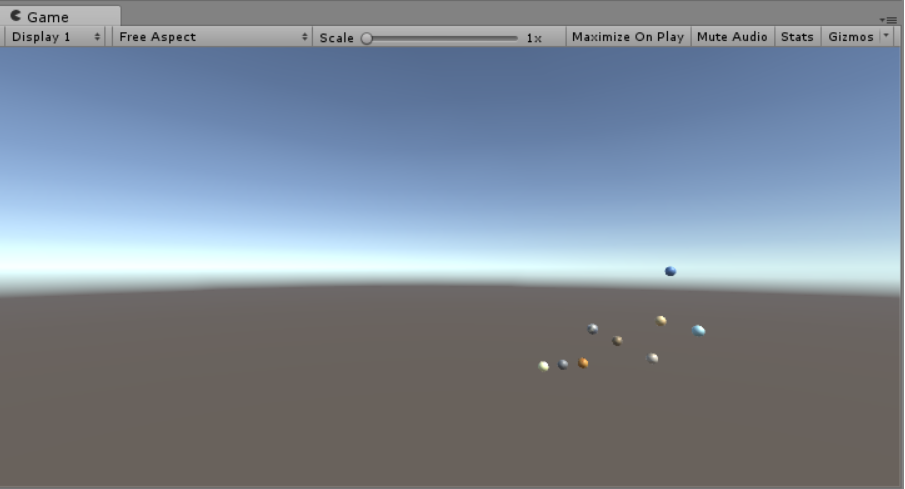总结

我们搭的一个简单的太阳系终于成功的跑起来啦，虽然真的是挺简单的，很多功能都没有实现，比如说，所有的星球都一样大，太阳不会发光，背景不好看等问题，但是总的来说，这个太阳系的雏形已经体现出来了，至于怎么把这个太阳系做得更加像我们的现实的太阳系，在我以后的博客里面，我会继续更新的（正经脸）。

补充

由于担心有些小伙伴看不懂英语，我在这里补充八大行星的中英文对照表（捂脸），以下的排名部分先后（笑）。

中文
英文

太阳
Sun

水星
Mercury

金星
Venus

地球
Earth

火星
Mars

木星
Jupiter

土星
Saturn

天王星
Uranus

海王星
Neptune

太阳系的贴图：https://pan.baidu.com/s/1dE9I6QX
第一篇博客就写到这啦。
展开全文unity3d
• 太阳系模拟，本来应该是按太阳系中太阳和行星的真实比例来实现的，后来，发现用真实比例，呈现出来的模拟结果很难看（太阳确实太大了，另外，大的行星和小的行星之间的比例相差也太大），所以，这次实验就没有按照...
      太阳系大概是什么样子，大家都知道的，这里就不多说了。太阳系模拟，本来应该是按太阳系中太阳和行星的真实比例来实现的，后来，发现用真实比例，呈现出来的模拟结果很难看（太阳确实太大了，另外，大的行星和小的行星之间的比例相差也太大），所以，这次实验就没有按照真实的数据比例来进行模拟啦。
先来看看成品图：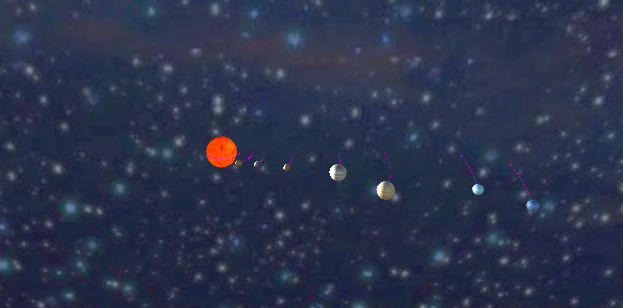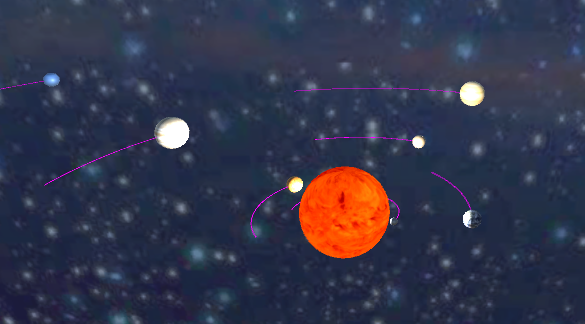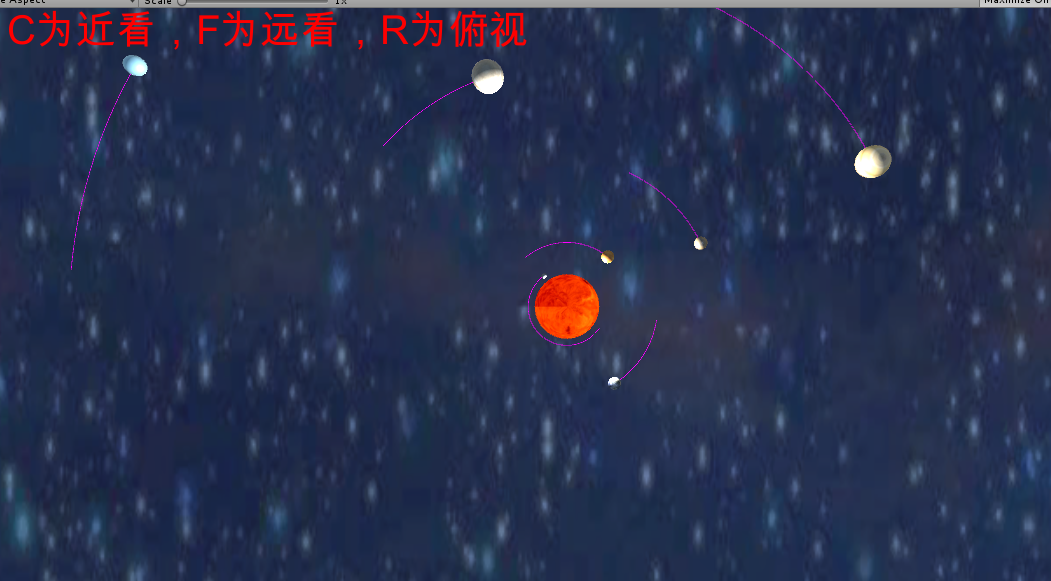下面，就看一下怎么弄吧

首先，就先下载好太阳和各行星的贴图：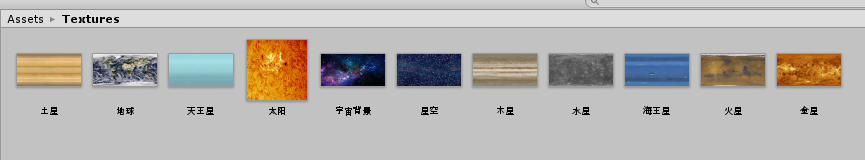并且用这些贴图来弄好太阳和各行星的prefabs：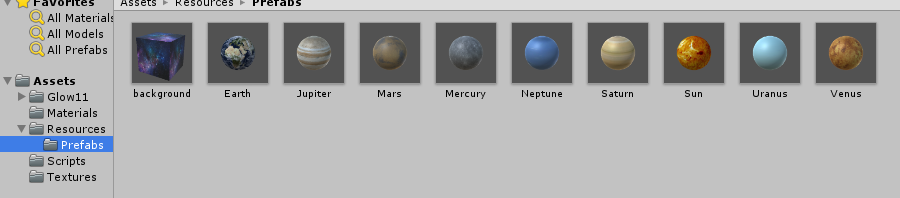接下来，就是代码实现了。

首先，就是导演类（单例模式，一个游戏只有一个导演）和场景控制接口，这两个就没什么可说的啦。

using System.Collections;
using System.Collections.Generic;
using UnityEngine;

public class SSDirector : System.Object {
//singlton instance
private static SSDirector _instance;

public IScenesController currentScenceController { get; set; }

public static SSDirector getInstance()
{
if(_instance == null)
{
_instance = new SSDirector();
}
return _instance;
}

public int getFPS()
{
return Application.targetFrameRate;
}

public void setFPS(int fps)
{
Application.targetFrameRate = fps;
}
}

using System.Collections;
using System.Collections.Generic;
using UnityEngine;

public interface IScenesController
{
}

然后，就是实现整个场景的控制器了。这里就不多说什么，看代码和注释胜过很多语言表达。

using System.Collections;
using System.Collections.Generic;
using UnityEngine;

public class Controller : MonoBehaviour, IScenesController {
private Transform[] planets = new Transform;
//行星名字，用于辅助后面资源导入
private string[] planets_name = { "Mercury", "Venus", "Earth", "Mars", "Jupiter", "Saturn", "Uranus", "Neptune" };
//三个摄影机，用来处理三个不同的拍摄视角
private GameObject ca_0;
private GameObject ca_1;
private GameObject ca_2;

//行星的初始位置，其实也可以看作是公转半径比例啦
Vector3[] position =
{
new Vector3(6, 0, 0),
new Vector3(10, 0, 0),
new Vector3(14, 0, 0),
new Vector3(23, 0, 0),
new Vector3(38, 0, 0),
new Vector3(53, 0, 0),
new Vector3(77, 0, 0),
new Vector3(90, 0, 0)
};
//各行星的公转法平面倾角
Vector3[] includedAngle = {
new Vector3 (0, 1, Mathf.Tan(Mathf.PI/180*7)),
new Vector3 (0, 1, Mathf.Tan(Mathf.PI/180*3.4f)),
new Vector3 (0, 1, 0),
new Vector3 (0, 1, Mathf.Tan(Mathf.PI/180*1.9f)),
new Vector3 (0, 1, Mathf.Tan(Mathf.PI/180*1.3f)),
new Vector3 (0, 1, Mathf.Tan(Mathf.PI/180*2.5f)),
new Vector3 (0, 1, Mathf.Tan(Mathf.PI/180*0.8f)),
new Vector3 (0, 1, Mathf.Tan(Mathf.PI/180*1.8f)),
};
//公转速率和自转速率。公转的胡乱编的，自转倒是比较真实
float[] gongZhuan = { 40.0f, 16.0f, 10.0f, 8.0f, 6.0f, 8.0f, 5.0f, 6.0f };
float[] ziZhuan = { 6.1f, -1.5f, 360f, 350f, 878f, 844.5f, -500.5f, 540.5f };

Transform Sun;//太阳组件，作为所有行星的父亲
//两个背景。至于为什么两个，就为了多个视角，然后，技术不够，只能用这种方法来实现背景
Transform background1;
Transform background2;
//导演的设定
void Awake()
{
SSDirector director = SSDirector.getInstance();
director.setFPS(60);
director.currentScenceController = this;

}
//生成transform组件的辅助函数
Transform createTransform(Vector3 position, string name)
{
Transform transform = Instantiate<Transform>(Resources.Load<Transform>("prefabs/" + name), position, Quaternion.identity);
return transform;
}
//资源导入
{   //导入太阳
Sun = createTransform(Vector3.zero, "Sun");
Sun.name = "Sun";
//导入背景
background1 = createTransform(new Vector3(0, -100, 500), "background");
background1.name = "Background";
background1.localScale += new Vector3(2000, 1000, 100);

background2 = createTransform(new Vector3(0, -500, 0), "background");
background2.name = "tBackground";
background2.localScale += new Vector3(2000, 2, 2000);
//导入各个行星
for (int i = 0; i < 8; i++)
{
planets[i] = createTransform(position[i], planets_name[i]);
planets[i].transform.parent = Sun.transform;
planets[i].name = planets_name[i];
//用来加尾巴的。不过，有一些属性我试过改了，呈现不出来，就干脆，原始这样子吧
trail.startWidth = 0.1f;
}
}

// 设置初始化视角为远看
void Start () {
ca_0 = GameObject.Find("Camera");
ca_0.SetActive(true);
ca_1 = GameObject.Find("Camera1");
ca_1.SetActive(false);
ca_2 = GameObject.Find("Camera2");
ca_2.SetActive(false);
}

// Update is called once per frame
void Update () {
//每个行星的自转和公转处理
for(int i = 0; i < 8; i++)
{
planets[i].RotateAround(Sun.position, includedAngle[i], gongZhuan[i] * Time.deltaTime);
planets[i].Rotate(Vector3.up * ziZhuan[i] * Time.deltaTime);
}
//获取键盘输入来得到观察的三个视角。C是近处，F是远处，R是俯视
if(Input.GetKeyDown(KeyCode.C))
{
ca_0.SetActive(false);
ca_1.SetActive(true);
ca_2.SetActive(false);
}
if(Input.GetKeyDown(KeyCode.F))
{
ca_1.SetActive(false);
ca_0.SetActive(true);
ca_2.SetActive(false);
}
if(Input.GetKeyDown(KeyCode.R))
{
ca_2.SetActive(true);
ca_1.SetActive(false);
ca_0.SetActive(false);
}
}
//为使用添加一点说明
void OnGUI()
{
GUIStyle fStyle = new GUIStyle();
fStyle.fontSize = 40;
fStyle.normal.textColor = Color.red;//红色比较容易看清
GUI.Label(new Rect(0, 0, 200, 50), "视角：C为近看，F为远看，R为俯视", fStyle);
}
}


代码实现完之后，也得对场景做一下处理，也很简单，就是把一个空对象和三个摄像头加上去，调整好它们的位置就好了。这里的话，为了观察方便，我三个摄像头都加了一个平行光，将光的强度调到了0.1。然后，在太阳的位置（就是Vector（0，0，0））上，增加一个点光，模拟太阳发出来的光线。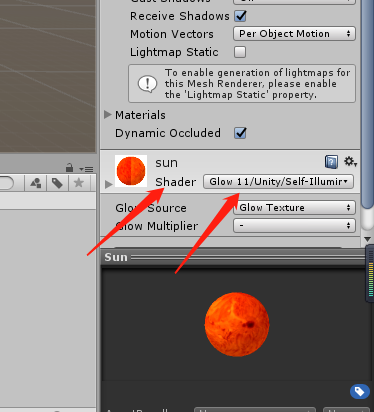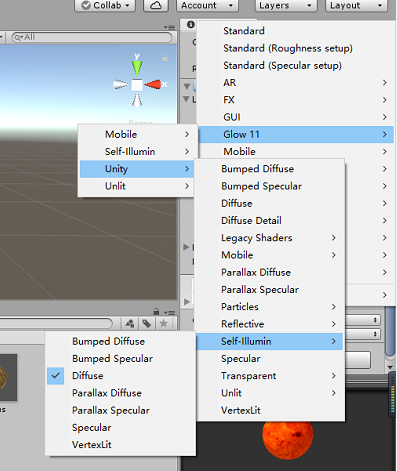之后，太阳看起来就是发亮的样子了。至此，这个太阳系就模拟地差不多了。
展开全文Unity3D C# 脚本
• 游戏对象运动的本质是什么？游戏对象的运动，在本质上是在离散的时间点上，物体按照物理引擎说运算出的，呈现出不同的位置。...方法二：collider 的gravity 模拟类似于上面，但云加速运动部分采用colli...
游戏对象运动的本质是什么？游戏对象的运动，在本质上是在离散的时间点上，物体按照物理引擎说运算出的，呈现出不同的位置。请用三种方法以上方法，实现物体的抛物线运动。（如，修改Transform属性，使用向量Vector3的方法…）方法一：矢量叠加法众所周知，一个抛物运动由水平方向的匀速和竖直方向的匀加速运动构成。方法二：collider 的gravity 模拟类似于上面，但云加速运动部分采用collider组件自带的gravity功能。方法三：transform.position 描述表达式法写一个程序，实现一个完整的太阳系， 其他星球围绕太阳的转速必须不一样，且不在一个法平面上。实现思路：利用transform的rotate、lookat、等方法，看上去像但并不真实的模拟出太阳系的运动。另一种实现思路：使用万有引力定律和牛顿第二运动定律，列出物体动力学方程。第二种方法的优点：可以模拟出水星的章动（椭圆周期运动上叠加小周期简谐振动）、各行星的椭圆轨道、冥王星和海王星的引力影响轨道。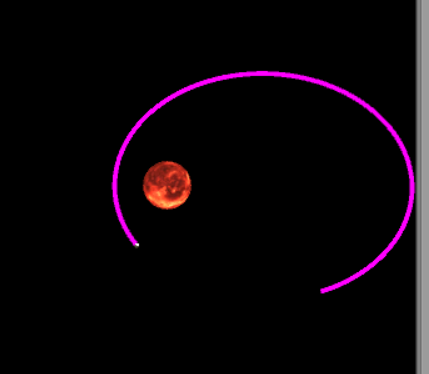引力模拟代码如下using System.Collections;
using System.Collections.Generic;
using UnityEngine;

public class GravitySimulate : MonoBehaviour {
public List<GameObject> graviEffectWith;
public Vector3 TotalGravitationalForce;
// Use this for initialization
void Start () {
//    this.GetComponent<PhysicalModel>().speed=startsetspeed();
}
public Vector3 startsetspeed()
{
this.GetComponent<PhysicalModel>().speed.z = Mathf.Sqrt(graviEffectWith.GetComponent<PhysicalModel>().mass /
Vector3.Distance(this.transform.position, graviEffectWith.transform.position)
);
return new Vector3(0, 0, this.GetComponent<PhysicalModel>().speed.z);
}
public void setSpeed(Vector3 input)
{
this.GetComponent<PhysicalModel>().speed = input;
}
// Update is called once per frame
void FixedUpdate () {
TotalGravitationalForce.Set(0, 0, 0);
foreach (GameObject i in graviEffectWith)
{

TotalGravitationalForce += -(i.GetComponent<PhysicalModel>().mass) /
(Vector3.Distance(this.transform.position, i.transform.position) * Vector3.Distance(this.transform.position, i.transform.position) * Vector3.Distance(this.transform.position, i.transform.position))
* (this.transform.position - i.transform.position);
}
this.GetComponent<PhysicalModel>().speed += TotalGravitationalForce*Time.deltaTime;
this.transform.position+=(this.GetComponent<PhysicalModel>().speed)*Time.deltaTime;
}
}
我们可以看到，简单的使用牛顿第二定律就可以画出真实的椭圆轨道。然后我们用一个PhysicalModel组件存储一些物理数据，此处略过在gameController里，我们储存了九大行星的数据（重点是距离、黄道交角）using System.Collections;
using System.Collections.Generic;
using UnityEngine;

public class SolarController : MonoBehaviour {
public GameObject SunPrefabs;
public GameObject Sun;
public GameObject[] SolarPlanetPrefabs;
public GameObject[] SolarPlanet;

public GameObject MoonPrefab;
public GameObject Moon;
Quaternion q;
// Use this for initialization
void Awake () {
SolarPlanet = new GameObject;
Sun = GameObject.Instantiate(SunPrefabs);
for (int i=0; i < SolarPlanetPrefabs.Length; ++i)
{
SolarPlanet[i] = GameObject.Instantiate(SolarPlanetPrefabs[i]);
//    SolarPlanet[i].GetComponent<GravitySimulate>().startsetspeed();
}
/*此处初始化行星*/
SolarPlanet.GetComponent<PhysicalModel>().SetPara(0.0553f,0.383f,3.87f);//质量半径距离
SolarPlanet.GetComponent<PhysicalModel>().SetPara(0.815f,0.950f,7.23f);
SolarPlanet.GetComponent<PhysicalModel>().SetPara(1,1,10);
SolarPlanet.GetComponent<PhysicalModel>().SetPara(0.107f, 0.532f, 15.24f);

//   SolarPlanet.GetComponent<PhysicalModel>().SetPara(95.159f,9.14f,52.03f);
// SolarPlanet.GetComponent<PhysicalModel>().SetPara(317.83f,10.97f,95.39f);
//   SolarPlanet.GetComponent<PhysicalModel>().SetPara(14.536f,3.98f,191.8f);
//Sun.GetComponent<PhysicalModel>().SetPara(332837f, 109.25f, 0);
Sun.GetComponent<PhysicalModel>().SetPara(33237f, 10.25f, 0);

/*下面设定初始速度*/
for (int i = 0; i < SolarPlanetPrefabs.Length; ++i)
{
SolarPlanet[i].GetComponent<GravitySimulate>().setSpeed(planetspeed(i));
SolarPlanet[i].GetComponent<selfRotate>().setRotateAxis(selfrotateAxis(i));
}

/*下面设定月球相关*/
Moon = Instantiate(MoonPrefab);
Moon.GetComponent<PhysicalModel>().GetComponent<PhysicalModel>().SetPara(0.18f, 0.1f, 10 + 0.0256f);
Moon.GetComponent<GravitySimulate>().setSpeed(Moon.GetComponent<GravitySimulate>().startsetspeed()+SolarPlanet.GetComponent<GravitySimulate>().startsetspeed());

}
Vector3 planetspeed(int index)//这个函数用来存储各种行星的初始速度
{
Vector3 theplanetspeed=SolarPlanet[index].GetComponent<GravitySimulate>().startsetspeed();

switch (index)
{
case 0:
q = Quaternion.AngleAxis(7.01f,new Vector3(1,0,0));
break;
case 1:
q = Quaternion.AngleAxis(3.39f, new Vector3(1, 0, 0));
break;
case 2:
q = Quaternion.AngleAxis(0.00f, new Vector3(1, 0, 0));
break;
case 3:
q = Quaternion.AngleAxis(1.85f, new Vector3(1, 0, 0));
break;
case 4:
q = Quaternion.AngleAxis(1.31f, new Vector3(1, 0, 0));
break;
case 5:
q = Quaternion.AngleAxis(2.49f, new Vector3(1, 0, 0));
break;
case 6:
q = Quaternion.AngleAxis(0.77f, new Vector3(1, 0, 0));
break;
case 7:
q = Quaternion.AngleAxis(1.77f, new Vector3(1, 0, 0));
break;
case 8:
q = Quaternion.AngleAxis(1.85f, new Vector3(1, 0, 0));
break;
}
Debug.Log(q * theplanetspeed);
return (q*theplanetspeed);
}

Vector3 selfrotateAxis(int index)//这个函数用来存储各种行星的初始自传角
{

switch (index)
{
case 0:
q = Quaternion.AngleAxis(28f, new Vector3(1, 0, 0));
break;
case 1:
q = Quaternion.AngleAxis(177f, new Vector3(1, 0, 0));
break;
case 2:
q = Quaternion.AngleAxis(23.45f, new Vector3(1, 0, 0));
break;
case 3:
q = Quaternion.AngleAxis(23.98f, new Vector3(1, 0, 0));
break;
case 4:
q = Quaternion.AngleAxis(3.08f, new Vector3(1, 0, 0));
break;
case 5:
q = Quaternion.AngleAxis(26.73f, new Vector3(1, 0, 0));
break;
case 6:
q = Quaternion.AngleAxis(97.92f, new Vector3(1, 0, 0));
break;
case 7:
q = Quaternion.AngleAxis(28.8f, new Vector3(1, 0, 0));
break;
case 8:
q = Quaternion.AngleAxis(1.85f, new Vector3(1, 0, 0));
break;
}
Debug.Log(q * new Vector3(0, 1, 0));
return (q * new Vector3(0,1,0));
}

}
在处理黄道交角时，我们用到了四元数与矩阵的积。高中物理知识告诉我们，调节物体的初速度就可以控制这个行星轨道的一切形状。比如，在黄道面上初速度不沿同心圆切线，那么这是个椭圆轨道。如果有垂直于黄道面的分速度，那么这个轨道将和黄道面异面！！！！接下来还做了行星的自转，但有些bug，就先不放上来了这个项目我会持续完善的，下一步时添加所有的行星、小行星带项目地址：https://github.com/kotomineshiki/cosmos.git
展开全文• Unity模拟太阳系，利用键盘鼠标交互。如果想在移动平台上用请更改输入响应。 包含太阳、水星，金星，地球，彗星。星球都有自转公转，貌似彗星无自转。 彗星轨迹为椭圆。 提供了WASD,滚轮，双击，右键交互。Unity
• unity3d 太阳系模型 地球围绕太阳转 月亮围绕地球转
• 本代码是基于Unity 3D太阳系模型，实现了太阳系，可以通过鼠标进行相关操作
• Unity 小项目：模拟太阳系 太阳系是以太阳为中心，和所有受到太阳的引力约束天体的集合体。包括八大行星（由离太阳从近到远的顺序：水星、金星、地球、火星、木星、土星、天王星、海王星 ）、以及至少173颗...
Unity 小项目：模拟太阳系

太阳系是以太阳为中心，和所有受到太阳的引力约束天体的集合体。包括八大行星（由离太阳从近到远的顺序：水星、金星、地球、火星、木星、土星、天王星、海王星 ）、以及至少173颗已知的卫星、5颗已经辨认出来的矮行星和数以亿计的太阳系小天体。

文章目录Unity 小项目：模拟太阳系1. 准备资源2. 布置场景3. 编辑脚本
1. 准备资源
由于太阳系的卫星以及小天体数目庞大，在本项目中，只模拟太阳和八大行星，以及地球的卫星月球。首先在网上搜索太阳系贴图，这里需要提醒一下大家，搜索的贴图不能是星球的照片，比如这种：否则覆盖到球体上就有黑色部分，会显得很奇怪。下面是在网上找到的所有贴图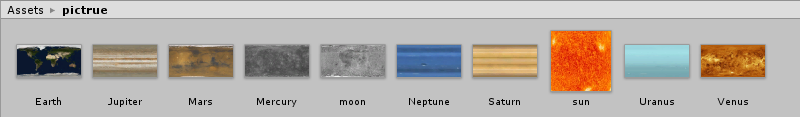然后就要制作Material了。八大行星和月球的Material比较简单，而太阳和它们不一样，太阳是一个恒星，它本身可以发光，这才照亮了太阳系中其他天体。所以在太阳的Material中，我们修改其Shader为如图所示的Self-Illumin，即自发光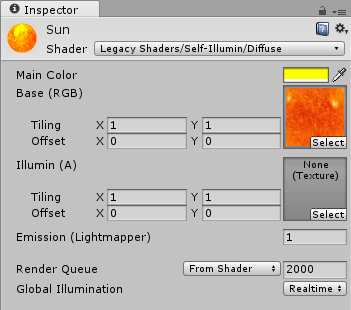下图是准备好的Material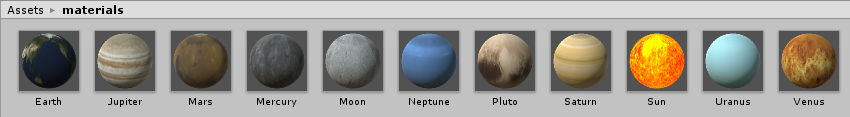2. 布置场景
建立十个球体，分别表示太阳，八大行星和月亮。按照大致的比例调整各个星球的大小距离即可。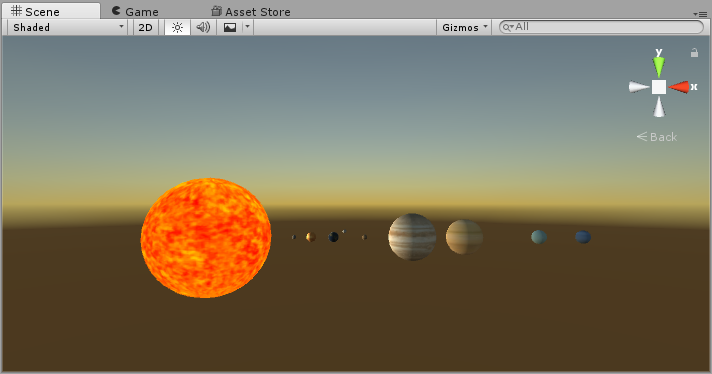在太阳系中，太阳是唯一光源，删除Directional Light后会显得比较合乎实际，但是我们会发现，场景中只有太阳是亮的，它并没有发出光照亮其他天体，所以我们在太阳中添加子物体Point Light，调整其亮度和范围。然后将Main Camera的Clear Flags属性改为Solid Color，并且将背景色改为黑色，这样运行起来，我们的太阳系就仿佛在浩瀚的宇宙中。
3. 编辑脚本
实现各天体公转、自转的代码比较简单。
using System.Collections;
using System.Collections.Generic;
using UnityEngine;

public class Rotate : MonoBehaviour {

public Transform origin;	//各天体公转的圆心
public float gspeed;		//公转速度
public float zspeed;		//自转速度
public float ry, rz;		//通过y轴、z轴调整公转的偏心率，使其不在同一平面公转

void Start() {

}

void FixedUpdate () {
Vector3 axis = new Vector3 (0, ry, rz);		//公转轴
this.transform.RotateAround (origin.position, axis, gspeed * Time.deltaTime);	//公转
this.transform.Rotate (Vector3.up * zspeed * Time.deltaTime);		//自转
}
}

将代码附到各天体上，分别设置好其公转速度，自转速度，公转偏心率**（错误：应该为公转平面的法线，偏心率是用于表示轨道形状，多谢评论指出）**，八大行星公转的圆心为太阳，月球的则是地球。最终的效果：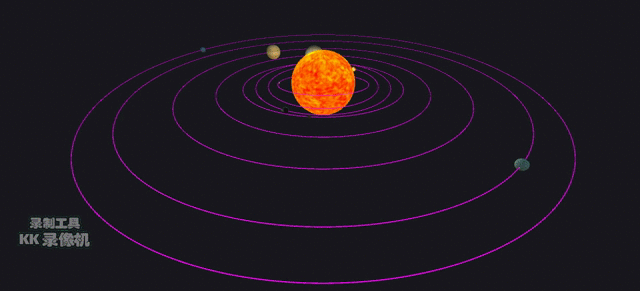上图是按照八大行星的实际偏心率所做出的效果，各天体轨道几乎在同一平面上，如果想要效果更明显一点，只需要稍稍修改各天体rz/ry的值就可以了。


展开全文unity 脚本
•unity3D学习 unity3d unity学习
• 这次要实现一个简单的太阳系，太阳本身自带光源，其他星球围绕太阳的转速不一样（越远的公转越慢），且不在一个法平面上。  首先，在场景中创建1个太阳和9大行星，然后将它们分别命名。9大行星的排列顺序是：...
• 写一个程序，实现一个完整的太阳系， 其他星球围绕太阳的转速必须不一样，且不在一个法平面上。 二.实验过程 创建如下结构 solar 里包括太阳和8大行星， 并且设置好距离和大小 在网上找到相应贴图 添加到assets 将...
• 实践要求：写一个程序，实现一个完整的太阳系，其他星球围绕太阳的转速必须不一样，并且不再一个法平面内。 法平面是指过空间曲线的切点，且与切线垂直的平面。要求不在一个法平面内，则在保证所有行星以及太阳在...
• unity3d 太阳 地球 月球 构成的建议太阳系模型 地球围绕太阳转 月亮围绕地球转 unity3d 3.0编制
• 最近沉迷一款游戏（我觉得叫他模拟软件更加合适），叫宇宙沙盒，是一款模拟我们大千宇宙的、烧电脑的模拟软件。刚入手就不做太多介绍，怕被真正的行家笑话。进入游戏，便是一个太阳系的模型。如图：我的灵感也是从这...unity Android
• 所有模型直径和轨道直径均按照1:10万km比例制作，所有行星公转角速度大致参照真实角速度绕太阳旋转，有卫星的基本也按照真实角速度绕中心天体旋转，通过滑杆控制前进后退速度和上下移动，滑动触屏旋转视角 ...unity3d android
•Unity3D
• 所有的资源（模型，贴图，脚本）全部开源放到了Github上，并配有详细文档。 https://github.com/youhengchan/Unity/blob/master/Unity%E6%A8%A1%E6%8B%9F%E5%A4%AA%E9%98%B3%E7%B3%BB.pdf ...
• Unity3D - 模拟太阳系 首先是制作太阳系中的每个行星，基本上都是先创建Sophere，然后改变起始位置，添加材质和贴图，这里就不赘述了。给每个行星创建材质包： 之后就是创建一个行星的移动脚本使得行星绕太阳公转...
...